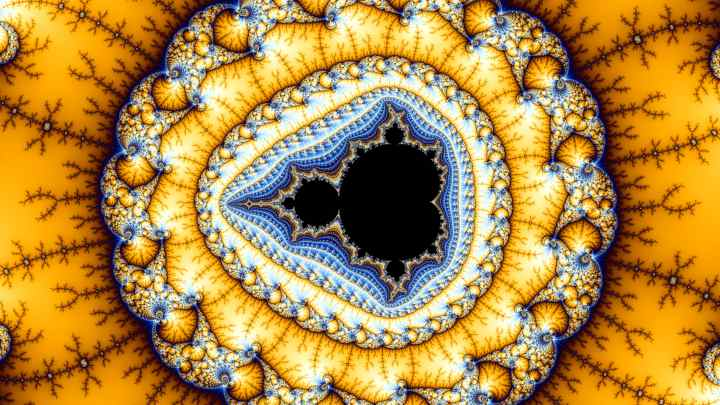# How to generate the Mandelbrot Set### How do you get from the formula to a picture?

It’s actually simple if you understand the meaning of the arrow that is used instead of =
It’s easiest when you see it in action, so let’s calculate the z->z²+c with c=1

z->z²+1     z always starts out as zero, so let’s add the 0 in.
0->0²+1    the result is 1.
Now we come to the arrow. It means that after calculating a first result, you take that result and use it as new value for z. So we change z to 1:
1->1²+1    result is 2. so for the next calculation (called “iteration”) we take 2 for z:
2->2²+1   result is 5, put back in for the next iteration:
5->5²+1   result is 26
26->26²+1    is 677
677->677²+1
458329->458329²+1  ….
There’s little point to continue this, it’s obvious the number will grow larger very fast.
The result of Mandelbrots formula is that for c=1 as starting value, it goes towards infinity.
All points that go towards infinity are NOT part of the Mandelbrot set.

But this doesn’t happen for all values. Let’s try a different example. how about c=-1
z->z²-1
1. Iteration 0->0²-1    result is -1, so we take -1 for z in the next iteration.
2. Iteration -1->-1²-1  result is  so z=0 for the 3. iteration)
3. Iteration 0->0²-1 (result is -1 again, using z=-1 for the 4. iteration)
4. Iteration -1->-1²-1
5. Iteration  0->0²-1
6. Iteration -1->-1²-1

So for c=-1 Mandelbrots formula doesn’t grow to infinity but oscillates between -1 and 0.
All points that never go to infinity are part of the Mandelbrot-Set.

##### So where’s the picture?

Well, it is hard work to calculate this by hand and it would take years to manually calculate a detailed picture. We actually have calculated just 2 pixels of a Mandelbrot-Set image.
A full-HD picture has 1920*1080 = 2.073.600 Pixels. So it’s no wonder the Mandelbrot -Set was only discovered after computers became fast enough.

For this simple explanation we’ll stick with the horizontal x-axis. For the vertical y-axis you need to understand imaginary numbers. To learn about them watch this video.
To visually play around with different values of c use this nice tool.

##### Iterations – how the Mandelbrot-Set becomes colorful

Remember the “iterations” from above?
The iterations are the number of repetitions needed to find out if a starting value goes to infinity.
As a rule of thumb, as soon as the result of a iteration is larger than 2, you can stop calculating, because all results over 2 will always go towards infinity and can never be a part of the Mandelbrot-Set. For c=1 the iteration-chain looked like this:
1. Iteration  0->0²+1
2. Iteration 1->1²+1   result is 2
3. Iteration 2->2²+1
With the second iteration it’s clear that the result will inevitably become larger and larger until infinity. So we can stop calculating and calculate the next pixel.

In the last picture, the center of the miniature Mandelbrot set has c value of -0.272162760214080041615297
Just to make clear why only computers have “the patience” to calculate these images, lets do the first few iterations of a single pixel in the center by hand:
1. Iteration:
0->0²+-0.272162760214080041615297
2. Iteration
-0.272162760214080041615297->-0.272162760214080041615297²+(–0.272162760214080041615297)
3.Iteration
-0.346235328261426871634995444->-0.346235328261426871634995444²+(–0.272162760214080041615297)
4.Iteration
-0.392041662750378062980754096877->-0.392041662750378062980754096877²+(-0.272162760214080041615297
5. Iteration … nope. That’s enough. But we need to do all 1024 iterations before it becomes clear that c=-0.272162760214080041615297  is actually part of the Mandelbrot-Set and will therefor be coloured black.

Now you’re prepared to appreciate a deep zoom – not only for it’s beauty, but also the incredible mathematical task that simulation is. Just for comparison, zooming from the whole visible universe down to a single Planck length is a zoom depth of 10^62. This videos final zoom depth is 10^96, the final coordinates are:
Real: -1.77983107263358987710609737625462554160612202082973721464269536941106123136243079619230058357360213932827
Imaginary:
0.0000768808104690890642867866831207682698968739265086118371576831945033925898525759232427462136212757994983490243570012245
Zoomdepth: 1.068E96
No matter who, when or where, when these coordinates are put into Mandelbrots formula, the result will be this exact zoom path and final picture.
Infinite complexity from a simple formula – simple mathematics creating the most common features in nature, they are embedded this simple formula: z->z²+c

And just in case you wonder – the current world record for deepest zoom is this with a final zoom depth of 10 to the power of 10002.
It took 2 full months to render.

Great freeware to explore the beauty of the Mandelbrot Set on PC right now: https://fractal.institute/old/fractal-software/kalles-fraktaler/